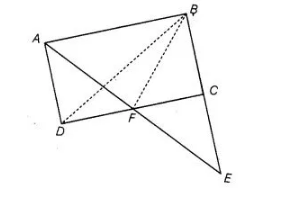# ABCD is a parallelogram in which BC`
Question:

ABCD is a parallelogram in which BC is produced to E such that CE = BC. AE intersects CD at FIf ar (ΔDFB) = 3 cm2, then find the area of the parallelogram ABCD.

Solution:

Given, $A B C D$ is a parallelogram and $C E=B C$ i.e., $C$ is the mid-point of $B E$.

Also, $\operatorname{ar}(\Delta D F B)=3 \mathrm{~cm}^{2}$

Now, $\triangle A D F$ and $\triangle D F B$ are on the same base $D F$ and between parallels $C D$ and $A B$. Then,

ar $(\triangle A D F)=\operatorname{ar}(\triangle D F B)=3 \mathrm{~cm}^{2}$ ...(i)

In $\triangle A B E$, by the converse of mid-point theorem,

$E F=A F$ [since, $C$ is mid-point of $B E] \ldots$ (ii)

In $\triangle A D F$ and $\triangle E C F$.

$\angle A F D=\angle C F E$ [vertically opposite angles]

$A F=E F$ [from Eq. (ii)]

and $\angle D A F=\angle C E F$

[since, $B E \| A D$ and $A E$ is transversal, then alternate interior angles are equal]

$\therefore \quad \triangle A D F \equiv \triangle F C F \quad$ [by ASA congruence rule]

Then, $\operatorname{ar}(\triangle A D F)=\operatorname{ar}(\triangle C F E)$

[since, congruent figures have equal area]

$\therefore \quad \operatorname{ar}(\Delta C F E)=\operatorname{ar}(\triangle A D F)=3 \mathrm{~cm}^{2} \quad$ [from Eq. (i)]...(iii)

Now, in $\triangle B F E, C$ is the mid-point of $B E$ then $C F$ is median of

$\triangle B F E$

$\therefore \quad a r(\Delta C E F)=\operatorname{ar}(\triangle B F C)$

[since, median of a triangle divides it into two triangles of equal area]

$\Rightarrow \quad$ ar $(\Delta B F C)=3 \mathrm{~cm}^{2}$ ...(iv)

Now, $\quad \operatorname{ar}(\Delta B D C)=\operatorname{ar}(\Delta D F B)+\operatorname{ar}(\Delta B F C)$

$=3+3=6 \mathrm{~cm}^{2}$ [from Eqs. (i) and (iv)]

We know that, diagonal of a parallelogram divides it into two congruent triangles of equal areas.

$\therefore \quad$ Area of parallelogram $A B C D=2 \times$ Area of $\triangle B D C$

$=2 \times 6=12 \mathrm{~cm}^{2}$

Hence, the area of parallelogram $A B C D$ is $12 \mathrm{~cm}^{2}$.